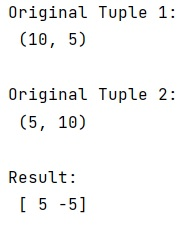# Elegant way to perform tuple arithmetic

Learn about the tuple arithmetic. We need to find the most elegant and concise way to perform tuple arithmetic.
Submitted by Pranit Sharma, on January 20, 2023

NumPy is an abbreviated form of Numerical Python. It is used for different types of scientific operations in python. Numpy is a vast library in python which is used for almost every kind of scientific or mathematical operation. It is itself an array which is a collection of various methods and functions for processing the arrays.

## Performing tuple arithmetic

Suppose that we are given two tuples and we need to perform some arithmetic operations on these tuples.

When it comes to fast operation, NumPy always helps us. Numpy has numerous arithmetic inbuilt functions that can help in fast computation.

Suppose that we subtract one tuple from another, we can use numpy.subtract() method and pass both the tuple as an input to this function.

Let us understand with the help of an example,

## Python code to demonstrate the elegant way to perform tuple arithmetic

```# Import numpy
import numpy as np

# Creating two tuples
t1 = (10,5)
t2 = (5,10)

# Display original tuples
print("Original Tuple 1:\n",t1,"\n")
print("Original Tuple 2:\n",t2,"\n")

# Subtracting t1 from t2
res = np.subtract(t1,t2)

# Display the result
print("Result:\n",res)
```

Output:What's New (MCQs)

Top Interview Coding Problems/Challenges!

IncludeHelp's Blogs

Languages: » C » C++ » C++ STL » Java » Data Structure » C#.Net » Android » Kotlin » SQL
Web Technologies: » PHP » Python » JavaScript » CSS » Ajax » Node.js » Web programming/HTML
Solved programs: » C » C++ » DS » Java » C#
Aptitude que. & ans.: » C » C++ » Java » DBMS
Interview que. & ans.: » C » Embedded C » Java » SEO » HR
CS Subjects: » CS Basics » O.S. » Networks » DBMS » Embedded Systems » Cloud Computing
» Machine learning » CS Organizations » Linux » DOS
More: » Articles » Puzzles » News/Updates